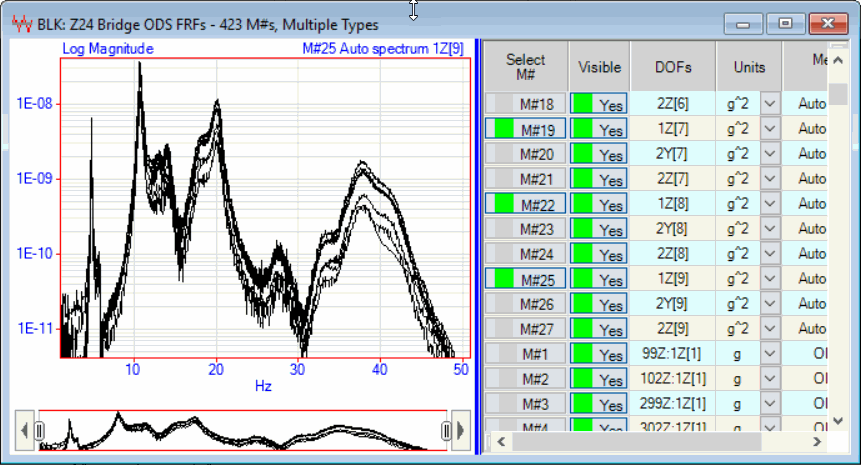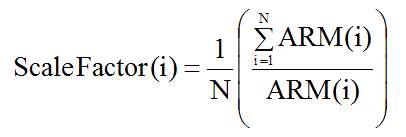Transform | Scale ODSFRFs

Re-scales ODSFRFs to correct for changes in the response levels between multiple Measurement Sets.

This command is only required when ODSFRF measurements are made using multiple Measurement Sets.

Overlaid Reference Auto Spectra

If there was any change of response levels between Measurement Sets, it can be observed by overlaying the Reference Auto Spectra from all Measurement Sets, as shown below.Overlaid Reference Auto Spectra From Multiple Measurement Sets.

Scale Factor

The ODSFRFs are re-scaled by first calculating an average Reference Auto Spectrum for all on the Measurement Sets.  Then, a scale factor for re-scaling the ODSFRFs in each Measurement Set is calculated using the following formula,where:

N = Number of Measurement Sets

ARM(i) = Average Magnitude of the Reference Auto spectrum for Measurement Set [ i ]

All ODSFRFs in Measurement Set [ i ] are multiplied by the Scale Factor ( i ).

• If the Line cursor is displayed, ARM(i) is calculated at the Line cursor position

• If the Band or Peak cursor is displayed, ARM(i) is calculated using all the samples in the band

• If no cursors are displayed, ARM(i) is calculated using all samples of each Reference Auto spectrum